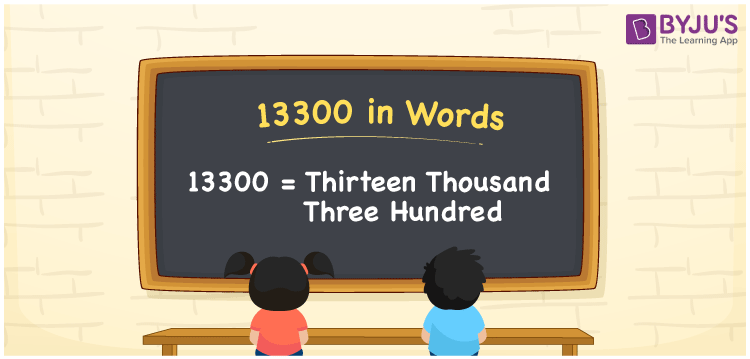# 13300 in Words

The number 13300 is represented by the word thirteen thousand three hundred. The number 13300 can be easily transformed into words using the place value of a number. As a result, the number names must be written using a place value table. For example, if you earned Rs. 13300 in a month, you can write “I earned Rs. Thirteen thousand three hundred in a month”. This article will show you how to write the number 13300 in English and how to spell it.

 13300 in Words: Thirteen Thousand Three Hundred. Thirteen Thousand Three Hundred in Numerical Form: 13300.

## 13300 in English Words## How to Write 13300 in Words?

The place value for the numeral 13300 is shown in the table below.

 Ten-thousands Thousands Hundreds Tens Ones 1 3 3 0 0

The extended form of the numeral 13300 is as follows.

= 1 × Ten thousand + 3 × Thousand + 3 × Hundred + 0 × Ten + 0 × One

= 1 × 10000 + 3 × 1000 + 3 × 100

= 10000 + 3000 + 300

= 13300

= Thirteen thousand three hundred

Hence, 13300 in words is thirteen thousand three hundred.

13300 in words – Thirteen thousand three hundred

Is 13300 an odd number? – No

Is 13300 an even number? – Yes

Is 13300 a perfect square number? – No

Is 13300 a perfect cube number? – No

Is 13300 a prime number? – No

Is 13300 a composite number? – Yes

## Frequently Asked Questions on 13300 in Words

Q1

### How to write 13300 in words?

13300 in words can be written as thirteen thousand three hundred.

Q2

### Simplify 14000 – 700, and express in words.

Simplifying 14000 – 700, we get 13300. Hence, 13300 in words is thirteen thousand three hundred.

Q3

### How to write thirteen thousand three hundred in numbers?

Thirteen thousand three hundred in numerals is 13300.

Test your Knowledge on 13300 in Words